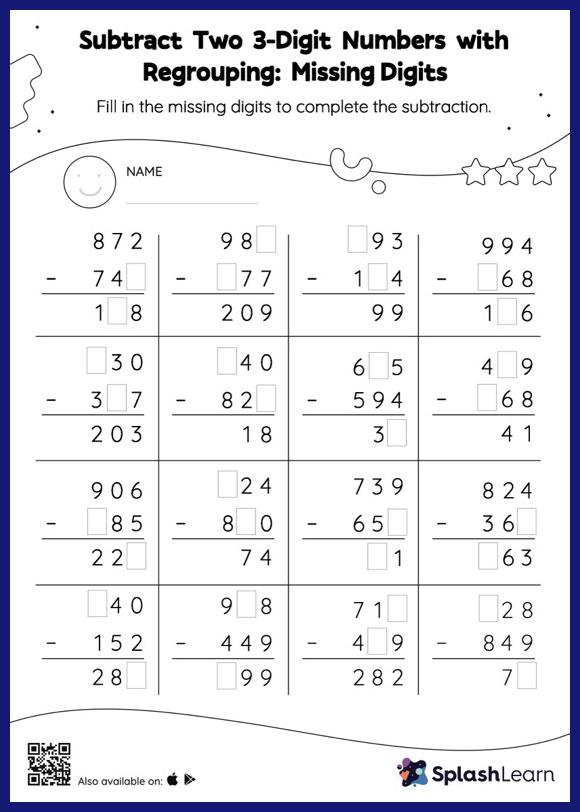# Subtract Two 3-Digit Numbers with Regrouping: Missing Digits Worksheet

Home > Subtract Two 3-Digit Numbers with Regrouping: Missing DigitsIn this worksheet, students must find the missing number using the count back method or the relationship between addition and subtraction. To solve subtract two 3-digit numbers with regrouping worksheet, they must also regroup the numbers. As the worksheet uses the column method, it is helpful in getting students toward higher accuracy, especially with bigger numbers and in scenarios where regrouping is required.